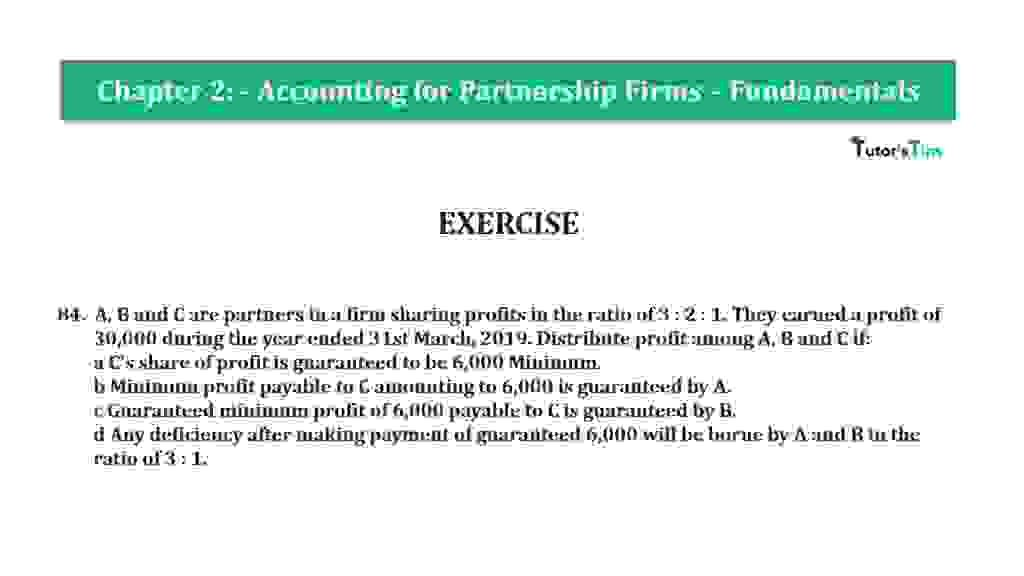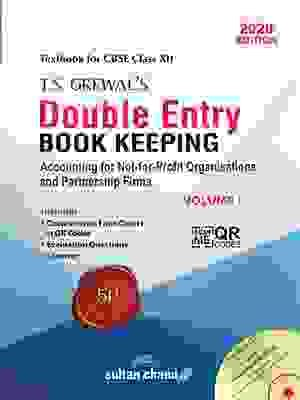# Question 84 Chapter 2 of +2-A – T.S. Grewal 12 Class Part – A Vol. 1Question 84 Chapter 2 of +2-A

84. A, B and C are partners in a firm sharing profits in the ratio of 3 : 2 : 1. They earned a profit of
30,000 during the year ended 31st March, 2019. Distribute profit among A, B and C if:
a C’s share of profit is guaranteed to be 6,000 Minimum.
b Minimum profit payable to C amounting to 6,000 is guaranteed by A.
c Guaranteed minimum profit of 6,000 payable to C is guaranteed by B.
d Any deficiency after making payment of guaranteed 6,000 will be borne by A and B in the ratio of 3 : 1.

The solution of Question 84 Chapter 2 of +2-A

:

Case A

 Balance Sheet (for the year ended 31st March 2019) Liabilities Amount Assets Amount By Profit and Loss A/c 30,000 To Profit Transferred to *2 A’s Capital A/c 14,400 B’s Capital A/c 9,600 C’s Capital A/c 6,000 30,000 30,000 30,000

Working Note: –

*1Calculation of distribution of Profits for the among the partners
Profit for year = 30,000
Profit-sharing ratio = 3: 2: 1
C is given a guarantee of a minimum profit of Rs 6,000
Anshu admitted for share = 1/6th share in profits

 A’s Share of Profit 30,000 X 3 6

A’s Share of Profit = 15,000

 B’s Share of Profit 30,000 X 2 6

B’s Share of Profit  = 10,000

 C’s Share of Profit 30,000 X 2 6

C’s Share of Profit = 5,000

C’s Actual Profit Share i. e. Rs 5,000 is less than his Minimum Guaranteed Profit i. e. Rs 6,000
Deficiency in C’s Profit Share= 6,000 − 5,000= Rs 1,000
This deficiency is to be borne by A and B in their profit sharing ratio i.e. 3: 2
This deficiency is to be borne by A and B in their profit sharing ratio i. e. 3: 2

 A’s Share of Profit = 1,000 X 3 5

A’s Share of Profit= 600

 B’s Share of Profit = 1,000 X 2 5

B’s Share of Profit= 400

Now, Final distributed among the partners

 A’s Share of Profit = 15,000 – 600 =14,400 B’s Share of Profit = 10,000 – 400 =9,600 C’s Share of Profit = 5,000 + 1,000 =6,000

Case B

 Balance Sheet (for the year ended 31st March 2019) Liabilities Amount Assets Amount By Profit and Loss A/c 30,000 To Profit Transferred to *2 A’s Capital A/c 14,000 B’s Capital A/c 10,000 C’s Capital A/c 6,000 30,000 30,000 30,000

C’s Actual Profit Share i.e. Rs 5,000 is less than his Minimum Guaranteed Profit i. e. Rs 6,000
Deficiency in C’s Profit Share = 6,000 − 5,000 = Rs 1,000
This deficiency is to be borne by A

Now, Final distributed among the partners

 A’s Share of Profit = 15,000 – 1,000 =14,000 B’s Share of Profit = 10,000 – 0 =10,000 C’s Share of Profit = 5,000 + 1,000 =6,000

Case C

 Balance Sheet (for the year ended 31st March 2019) Liabilities Amount Assets Amount By Profit and Loss A/c 30,000 To Profit Transferred to *2 A’s Capital A/c 15,000 B’s Capital A/c 9,000 C’s Capital A/c 6,000 30,000 30,000 30,000

C’s Actual Profit Share i.e. Rs 5,000 is less than his Minimum Guaranteed Profit i. e. Rs 6,000
Deficiency in C’s Profit Share = 6,000 − 5,000 = Rs 1,000

This deficiency is to be borne by B

Now, Final distributed among the partners

 A’s Share of Profit = 15,000 – 0 =15,000 B’s Share of Profit = 10,000 – 1,000 =9,000 C’s Share of Profit = 5,000 + 1,000 =6,000

Case  d

 Balance Sheet (for the year ended 31st March 2019) Liabilities Amount Assets Amount By Profit and Loss A/c 30,000 To Profit Transferred to *2 A’s Capital A/c 14,250 B’s Capital A/c 9,750 C’s Capital A/c 6,000 30,000 30,000 30,000

C’s Actual Profit Share i.e. Rs 5,000 is less than his Minimum Guaranteed Profit i. e. Rs 6,000
Deficiency in C’s Profit Share= 6,000 − 5,000= Rs 1,000
This deficiency is to be borne by A and B in ratio i.e. 3: 1

 A’s Share of Profit = 1,000 X 3 4

A’s Share of Profit= 750

 B’s Share of Profit = 1,000 X 2 4

B’s Share of Profit= 250

 A’s Share of Profit = 15,000 – 750 =14,250 B’s Share of Profit = 10,000 – 250 =9,750 C’s Share of Profit = 5,000 + 1,000 =6,000

Also, Check out the solved question of previous Chapters: –

### T.S. Grewal’s Double Entry Book Keeping +2 (Vol. I: Accounting for Not-for-Profit Organizations and Partnership Firms)

• Chapter No. 1 – Financial Statement of Not-For-Profit Organisations
• Chapter No. 2 – Accounting for Partnership Firms – Fundamentals
• Chapter No. 3 – Goodwill: Nature and Valuation
• Chapter No. 4 – Change in Profit-Sharing Ratio Among the Existing Partners
• Chapter No. 5 – Admission of a Partner
• Chapter No. 6 – Retirement/Death of a Partner
• Chapter No. 7 – Dissolution of a Partnership Firm

### T.S. Grewal’s Double Entry Book Keeping (Vol. II: Accounting for Companies)

• Chapter No. 1 – Financial Statements of a Company
• Chapter No. 2 – Financial Statement Analysis
• Chapter No. 3 – Tools of Financial Statement Analysis – Comparative Statements and Common- Size Statements
• Chapter No. 4 – Accounting Ratios
• Chapter No. 5 – Cash Flow StatementVol. I: Accounting for Not-for-Profit Organizations and Partnership Firms